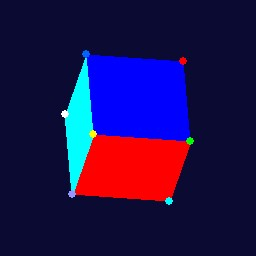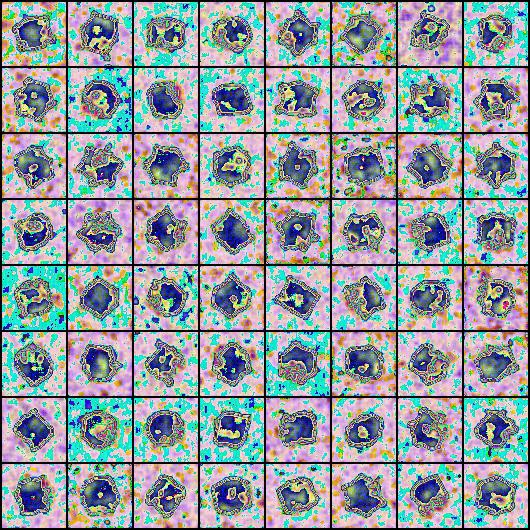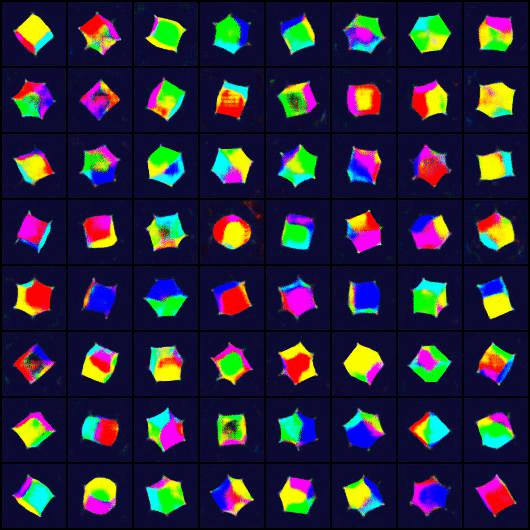# cLPR: A 3D Dataset for learning pose and rotation

Today I’m releasing the cLPR dataset, a dataset for doing work in 3D machine learning. The name stands for Cubes for Learning Pose and…

### cLPR: A 3D Dataset for learning pose and rotation

Today I’m releasing the cLPR dataset, a dataset for doing work in 3D machine learning. The name stands for Cubes for Learning Pose and Rotation. The goal is to provide a nice baseline dataset of colored 3D cubes in many different positions for testing machine learning algorithms whose goal is to learn about pose and rotation.

Download the dataset here:

https://bit.ly/2yeeA15

#### The Data

The dataset contains 32x32, 64x64, 128x128, 256x256 jpeg images of a cube (2d projections of the 3d object).The jpegs do not have any quality loss. The data looks like this:The cube in 3 different positions. The first and third image are showing 2 of the same sides (red and cyan).

#### The Labels

The labels are provided in the binary numpy file. The file is called `cube1–1.14.3–2018–06–10–12–30–10.npy`, generally `cubeN-x.xx.x-datetime.npy` where `x.xx.x` is the numpy version used to dump the file and `N` is the cube this data file is for. It contains every position and rotation of the nodes in a cube for all images in the dataset. For this dataset the structure is 250047 x 8 x 6, It’s POSITIONS x NODES x 6 numpy array, where the first 3 values of the last dimension (0,1,2) are x,y,z position and the last 3 values in the last dimention (3,4,5) are x,y,z rotation in radians. You can also just create whatever labels you want by modifying what the script stores in the `rotation_positions` array (`projector.py`, line 90).

So the structure looks like:

#### Making the Dataset

The dataset is produced using a package called `pygame` which is a package originally designed for making python games. The package is nice because it lets you easily draw and render objects in 2d or 3d. I used pygame because I wanted the code to be easy enough for someone with limited graphics experience and basic knowledge of matrix multiplication to understand.

The code used to generate the dataset is on github. Let’s go over how that code works at a high level.

#### Step 1 Create a Wireframe

First we create a wireframe of the cube. This wireframe consists of nodes, edges, and faces. The nodes are points in space, each represented with x,y,z coordinates. We store all the nodes in an 8x3 numpy array. Edges are two nodes (the indices of the nodes in the numpy array). Faces are 4 nodes connected together (again the indices of the nodes in the numpy array).

You can create a wireframe like this:

I use this to create 8 nodes, 6 faces. You don’t actually need to specify edges, just nodes and faces. You can test a cube wireframe creation; run `python wireframe.py` script included in the repository:

For this wireframe class the only outside dependency for `wireframe.py` is `numpy`. This script will create a cube and print the nodes, edges, and faces.

#### Step 2 Generate Rotations

Then I generate a set of rotations I’d like to apply in 3D to the nodes of the cube (the corners of the cube). To generate the rotations I just loop through all possible combinations of rotations from 0-6.3 radians(close to 2π radians but not exactly perfect).

The above snippet will generate roughly 360 degrees, 2π of rotation in radians. Then every frame/iteration of pygame we will grab one of these rotations and display it.

#### Step 3 Render the Wireframe

I render the wireframe using pygame’s functions to draw polygons. The function takes a series of edges (2 points, [(x,y,z), (x,y,z)]) and draws a polygon on screen with that color in the order of the points and edges given. Below is a quick and dirty version of my code; the actual script is a bit more involved.

#### Step 4 Apply one Rotation and Re-Center the Cube

To apply a rotation to this cube I use a rotation matrix for the appropriate axis. So to rotate around the x axis I would use `create_rot_x` from `wireframe.py`. This function returns a matrix for doing rotations in the x axis for the number of radians we need. Then I just do a dot product in the `transform` function between the nodes of the wireframe and the rotation matrix we created. All this does is multiply the x,y,z positions of our nodes by the right numbers in the matrix such that the new positions are rotated by however many radians along x. I repeart the above process for x,y and z radians for this rotation. After this is done re-center the cube so its middle is around the center of the screen.

#### Step 5 Reset the cube nodes to their initial position

I store the initial position and reset it to that after every rotation+re-center+snapshot of the cube. This just makes it easier to reason about what the cube does on every iteration, move to exactly the rotation I give it. Moving from one rotation to another can also have problems with something called gimbal lock. It is a bit of a mind bender so I wanted to avoid it entirely.

#### Step 6 Repeat Steps 3–5

While I do this I screen shot the pygame screen after every rotation and store position information in a numpy array. During the process I write the images to disk and at the end I save a numpy array with all the nodes’ positions and rotations.

#### Final step

Visual inspection and programatic testing of labels. I play back the numpy file (the `-t` input option to `projector.py` does plyback of npy file) and watch that the rotations look good. Also run it through the `test_npy_positions` function (the `-n` input to `projector.py`) which compares it a against known correct values for the positions at every section. The explanations above are intended to give you a sense of how the code works, see the code on github for more details. The code is not perfect, neither is the dataset and there’s a lot of room to improve (i.e. adding color mappings to the labels to make tracking easier). Please feel free to make a pull request or hack around on your own. I would really appreciate it.

#### What can you do with this dataset?

One thing you can do is train variational autoencoders. Just to show an example of how to use this dataset I’ve provided two variational auto encoders (one classic, one convlutional). Both are bit, underwhelming … but the point is just to show an example, not to have a perfect model.

Below is a picture where each cell represents an area of the latent space I have sampled and then put through our decoder network to generate a cube:

Not all of these look like cubes, but hey a couple of them do! The code is in this notebook.

Don’t get discouraged. Generative models are notoriously hard and finicky, here’s an example of one of my failed model outputs:Failed (yet cool) cube outputs.

Here is the obligatory gif (snapshot at every epoch) of the vae model I made above training on a subsample of the data:GIF of training VAE

If you would like a much better example of autoencoders trying different distributions in the autoencoder please check out this paper (and accompanying github code), written by my friend Tim Davidson at Universiteit van Amsterdam and several of his colleagues. There is also a really cool paper on SO3 autoencoders using a variant of this dataset.

#### Conclusion

Thanks for making it to the end. If you find an issue with the dataset or any of the scripts please reach out to me; people are already using this data (pre-release) for research papers and projects so issues should be known, publicized, and debugged.

If you would like to cite this dataset you can do so:

`Yvan Scher (2018). cLPR Dataset. Brooklyn, NY: The Internet`
`OR`
`@misc{Scher:2018,author = "Yvan Scher",year = "2018",title = "cLPR Dataset",url = "https://bit.ly/2yeeA15",institution = "The Internet"}`
`OR`
`Just say you used the cLPR dataset and link back to this post :)`

I want to thank express my thanks to peter collingridge who has some good pygame tutorials. I have done other blogs with pygame if that’s something that interests you. If you want to stay up to date with projects and interesting stuff happening in graphics and ai you should subscribe to my newsletter: Generation Machine.

Cheers!